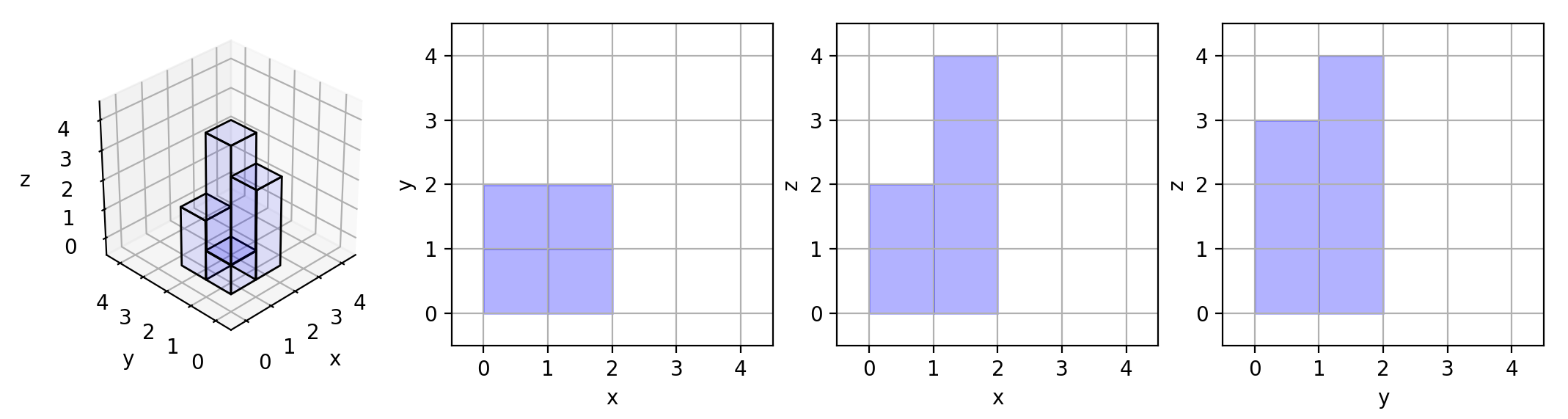## Algorithm

Problem Name: 883. Projection Area of 3D Shapes

You are given an `n x n` `grid` where we place some `1 x 1 x 1` cubes that are axis-aligned with the `x`, `y`, and `z` axes.

Each value `v = grid[i][j]` represents a tower of `v` cubes placed on top of the cell `(i, j)`.

We view the projection of these cubes onto the `xy`, `yz`, and `zx` planes.

A projection is like a shadow, that maps our 3-dimensional figure to a 2-dimensional plane. We are viewing the "shadow" when looking at the cubes from the top, the front, and the side.

Return the total area of all three projections.

Example 1:```Input: grid = [[1,2],[3,4]]
Output: 17
Explanation: Here are the three projections ("shadows") of the shape made with each axis-aligned plane.
```

Example 2:

```Input: grid = []
Output: 5
```

Example 3:

```Input: grid = [[1,0],[0,2]]
Output: 8
```

Constraints:

• `n == grid.length == grid[i].length`
• `1 <= n <= 50`
• `0 <= grid[i][j] <= 50`

## Code Examples

### #1 Code Example with Java Programming

```Code - Java Programming```

``````
class Solution {
public int projectionArea(int[][] grid) {
int sum = 0;

for (int i=0; i 0) {
sum++;
}

maxRow = Math.max(maxRow, grid[i][j]);
maxCol = Math.max(maxCol, grid[j][i]);
}

sum += maxCol + maxRow;
}

return sum;
}
}
``````
Copy The Code &

Input

cmd
grid = [[1,2],[3,4]]

Output

cmd
17

### #2 Code Example with Javascript Programming

```Code - Javascript Programming```

``````
const projectionArea = function(grid) {
let xy = 0, xz = 0, yz = 0
const m = grid.length, n = grid.length
for (let i = 0; i < m; i++) {
for(let j = 0; j < n; j++) {
if(grid[i][j]) xy++
}
}

for (let i = 0; i < m; i++) {
let tmp = 0
for(let j = 0; j < n; j++) {
tmp = Math.max(tmp, grid[i][j])
}
xz += tmp
}
for (let j = 0; j < n; j++) {
let tmp = 0
for(let i = 0; i < m; i++) {
tmp = Math.max(tmp, grid[i][j])
}
yz += tmp
}

return xy + yz + xz
};
``````
Copy The Code &

Input

cmd
grid = [[1,2],[3,4]]

Output

cmd
17

### #3 Code Example with Python Programming

```Code - Python Programming```

``````
class Solution:
def projectionArea(self, grid):
"""
:type grid: List[List[int]]
:rtype: int
"""
n = len(grid)
top = sum(grid[i][j] != 0 for i in range(n) for j in range(n))
front = sum(max(grid[i]) for i in range(n))
side = sum(max(grid[i][j] for i in range(n)) for j in range(n))
``````
Copy The Code &

Input

cmd
grid = []

Output

cmd
5

### #4 Code Example with C# Programming

```Code - C# Programming```

``````
using System;

namespace LeetCode
{
public class _0883_ProjectionAreaOf3DShapes
{
public int ProjectionArea(int[][] grid)
{
var N = grid.Length;

var result = 0;
for (int i = 0; i < N; i++)
{
int bestRow = 0, bestCol = 0;
for (int j = 0; j < N; j++)
{
if (grid[i][j] > 0) result++;
bestRow = Math.Max(bestRow, grid[i][j]);
bestCol = Math.Max(bestCol, grid[j][i]);
}
result += bestRow + bestCol;
}

return result;
}
}
}
``````
Copy The Code &

Input

cmd
grid = []

Output

cmd
5